Smartick is an advanced online program that teaches kids math and coding in only 15 min. a day

Dec28

# Complementary Angles: What Are They?

In today’s post, we are going to learn about what complementary angles are and their properties. After, we will look at some examples and complete a few exercises together.

Before we begin, we would recommend reviewing the types of angles that exist.

Index

## What Are Complementary Angles?

Two angles are complementary if the sum of the angles is equal to 90º (ninety degrees). For example, an angle that is 30º and an angle that is 60º are two complementary angles.

Since 90º is the measurement for a right angle, another way to tell if two angles are complementary is if, together, they form a right angle.

## Examples of Complementary Angles

Let’s represent some examples graphically.

### Two Different Complementary Angles

In this example, the measurements of the blue angle and the pink angle are different. However, when we add them together they equal 90º.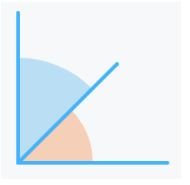### Two Equal Complementary Angles

We can see two 45º angles that together, equal 90º. Did you know, if two complementary angles are the same, then they both measure 45º, because 45º + 45º = 90º!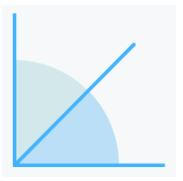### Two Different Angles That Are Not Complementary

In this example, we have a green angle and a purple angle, which together equal 180º,  so they are not complementary. When two angles added together equal 180º, then they are supplementary angles.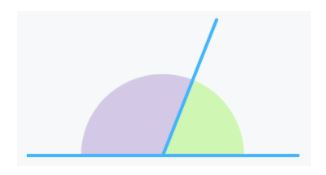So far, all of the angles that we have seen in the previous examples are together, in other words, they are consecutive.

Now we are going to see more examples of angles that are not consecutive.

### Two Equal Complementary Angles That Are Not Consecutive

Here we have two 45º angles that, although they are not together (they are not consecutive), they are still two complementary angles.### Two Different Complementary Angles That Are Not Consecutive

In this example, we can see two different angles that when joined together form a 90ºangle, in other words, they are complementary.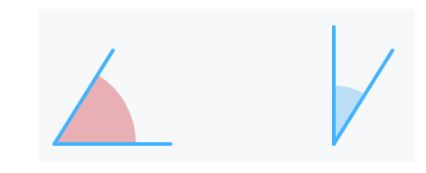## Practice with Complementary Angles in Smartick

In children’s daily Smartick sessions they are presented with geometry exercises that are adapted to their level. One of the types of angle activities that can be found is to determine if the angles that appear are complementary or not. Are you able to complete the following exercises?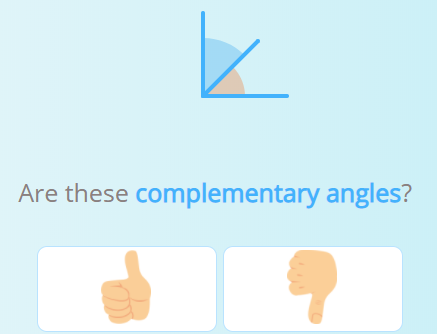Solution: YESSolution: NO

## Video: Relationships Between Angles

I hope that you have enjoyed today’s post.

Below is a non-interactive video tutorial about the relationships between angles. In this video, Eva and Zoe go to a bike shop and decorate the wheels on their new bicycles while learning about the relationships that exist between angles. Watch the video to decorate their bicycles with them!

If you would like to access interactive tutorials, to be solved by yourself, and to learn much more about primary school mathematics, register with Smartick to try it for free! We’re excited to learn with you!